# NCERT Solutions class 7 Maths Chapter-3 Exercise 3.1

NCERT Solutions Class-7 Maths chapter-3 data handling Exercise-3.1 is prepared by academic team of Physics Wallah all the questions of NCERT text book are solved step by step with proper and detail solutions explaining each and every questions. For More and additional questions of CBSE class 7 maths you can go to class 7 maths sections. NCERT class 7 Maths Solutions is the best way to enhanced your mathematics skill. And Physics Wallah practice worksheet & question bank will help you a lot.

## NCERT Solutions class 7 Maths Chapter-3 Data handling

### Solutions of Chapter Data handling Exercise-3.1

Question 1: Find the range of heights of any ten students of your class. Answer:

Let the heights (in cm) of 10 students of our class be 125, 127, 132, 133, 134, 136,138, 141, 144, 146

Highest value among these observations = 146

Lowest value among these observations = 125

Range = Highest value − Lowest value

= (146 − 125) cm

= 21 cm

Question 2: Organise the following marks in a class assessment, in a tabular form.

 4 6 7 5 3 5 4 5 2 6 2 5 1 9 6 5 8 4 6 7

(i) Which number is the highest?

(ii) Which number is the lowest?

(iii) What is the range of the data?

(iv) Find the arithmetic mean.

According to the question,

At first,

We will draw the frequency table of the given data.

Hence,

 Marks Tally marks Frequency 1 I 1 2 II 2 3 I 1 4 III 3 5 IIII 5 6 IIII 4 7 II 2 8 I 1 9 I 1

(i) Highest number = 9

(ii) Lowest number = 1

(iii) Range = (9 − 1) = 8

(iv) Sum of all the observations = 4 + 6 + 7 + 5 + 3 + 5 + 4 + 5 + 2 + 6 + 2

+ 5 + 1 + 9 + 6 + 5 + 8 + 4 + 6 + 7

= 100

Total number of observations = 20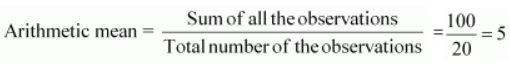Question 3:

Find the mean of the first five whole numbers.

First five whole numbers are 0, 1, 2, 3, and 4.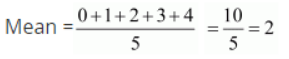Therefore, the mean of first five whole numbers is 2.

Question 4:

A cricketer scores the following runs in eight innings:

58, 76, 40, 35, 46, 45, 0, 100

Find the mean score.

Runs scored by the cricketer are 58, 76, 40, 35, 46, 45, 0, and 100.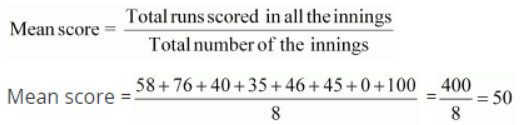Therefore, mean score is 50. Question 5:

Following table shows the points of each player scored in four games:

 Player Game 1 Game 2 Game 3 Game 4 A 14 16 10 10 B 0 8 6 4 C 8 11 Did not play 13

(i) Find the mean to determine A’s average number of points scored per game.

(ii) To find the mean number of points per game for C, would you divide the total points by 3 or by 4? Why?

(iii) B played in all the four games. How would you find the mean?

(iv) Who is the best performer?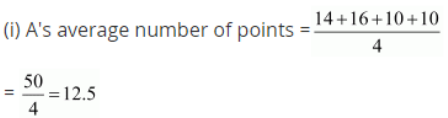(ii) To find the mean number of points per game for C, we will divide the total points by 3 because C played 3 games.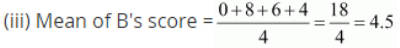(iv) The best performer will have the greatest average among all. Now we can observe that the average of A is 12.5 which is more than that of B and C. Therefore, A is the best performer among these three.

Question 6:

The marks (out of 100) obtained by a group of students in a science test are 85, 76, 90, 85, 39, 48, 56, 95, 81 and 75. Find the:

(i) Highest and the lowest marks obtained by the students.

(ii) Range of the marks obtained.

(iii) Mean marks obtained by the group.

The marks obtained by the group of students in a science test can be arranged in an ascending order as follows.

39, 48, 56, 75, 76, 81, 85, 85, 90, 95

(i) Highest marks = 95

Lowest marks = 39

(ii) Range = 95 − 39

= 56